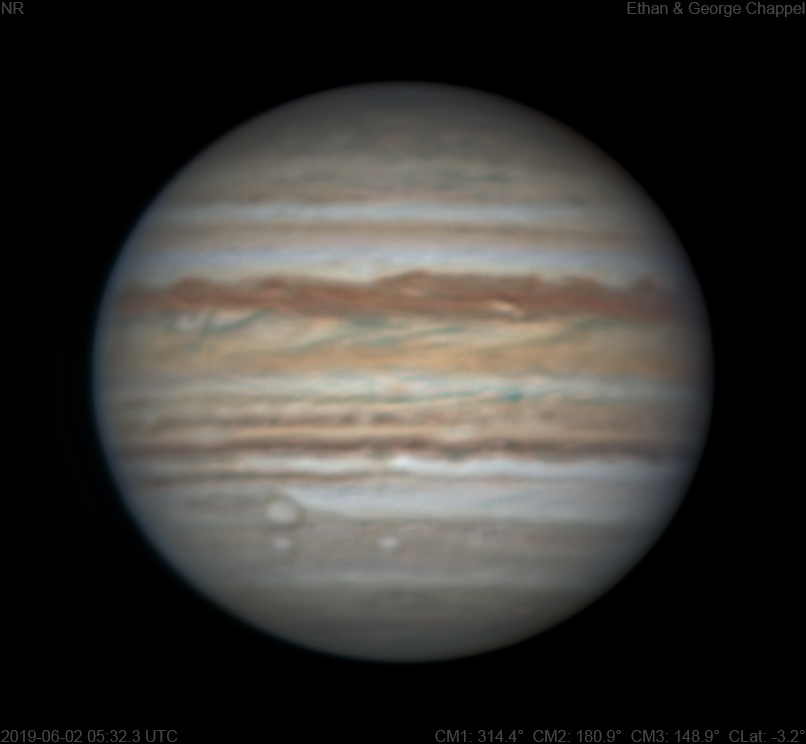# Jupiter 2019-06-02 05:32 UTC

CM1: 314.40°

CM2: 180.90°

CM3: 148.90°

CLat: -3.20°

Description

After a successful set of images with the 90mm Mak and no signs of clouds, we decided to take out the C14 while there was time. Turns out that was a really good decision since this is our best RGB image the apparition so far. However, it did end up being disturbed by a thin layer of clouds.

Notes:

• The North North Temperate Belt is showing hints of orange within its otherwise brown clouds.
• The North Temperate Belt is thin. The southern edge is faintly orange while the north is a thin dark line.
• There is a white oval on the NEBn at the central meridian.
• The North Equatorial Belt has a new rift past the CM.
• The Equatorial Zone, except for the southern edge, is still orange. However, there is a subtle orange smudge at the CM on the EZs.
• The South Equatorial Belt has a teal spot with a detached tail on its northern edge. I don't recall seeing anything with this color on Jupiter before.
• The SEBs is dark compared to the rest of the belt.
• There is a bright spot just off the SEBs on the CM.
• White Oval BA is rising with the STB Ghost trailing it.
• There is a subtle blue feature to the west of Oval BA. Is this an STB structured segment?
• The South Polar Red Spot is setting.

Equipment

ZWO ASI290MM

Celestron C14 EdgeHD

Logs
```FireCapture v2.6  Settings
------------------------------------
Observer=Ethan Chappel
Camera=ZWO ASI290MM
Filter=R
Profile=Jupiter
Diameter=45.80"
Magnitude=-2.60
CMI=308.9° CMII=175.4° CMIII=143.5°  (during mid of capture)
FocalLength=7900mm (F/28)
Resolution=0.08"
Filename=2019-06-02-0523_4-EC-R-Jup.ser
Date=2019_06_02
Start=05_22_26.648
Mid=05_23_26.653
End=05_24_26.658
Start(UT)=05_22_26.648
Mid(UT)=05_23_26.653
End(UT)=05_24_26.658
Duration=120.010s
Date_format=yyyy_MM_dd
Time_format=HH_mm_ss
LT=UT -6h
Frames captured=7204
File type=SER
Binning=no
Bit depth=8bit
Debayer=no
ROI=796x746
ROI(Offset)=0x0
FPS (avg.)=60
Shutter=14.01ms
Gain=416 (69%)
Gamma=50 (off)
Brightness=1 (off)
FPS=100 (off)
AutoGain=off
SoftwareGain=10 (off)
USBTraffic=40 (off)
AutoExposure=off
AutoHisto=75 (off)
HighSpeed=off
Histogramm(min)=0
Histogramm(max)=230
Histogramm=90%
Noise(avg.deviation)=2.79
AutoAlign=false
PreFilter=none
Limit=120 Seconds
Sensor temperature=35.3°C
Focuser position=3297
FireCapture v2.6  Settings
------------------------------------
Observer=Ethan Chappel
Camera=ZWO ASI290MM
Filter=G
Profile=Jupiter
Diameter=45.80"
Magnitude=-2.60
CMI=311.2° CMII=177.7° CMIII=145.7°  (during mid of capture)
FocalLength=7850mm (F/28)
Resolution=0.08"
Filename=2019-06-02-0527_2-EC-G-Jup.ser
Date=2019_06_02
Start=05_26_14.975
Mid=05_27_14.981
End=05_28_14.988
Start(UT)=05_26_14.975
Mid(UT)=05_27_14.983
End(UT)=05_28_14.992
Duration=120.013s
Date_format=yyyy_MM_dd
Time_format=HH_mm_ss
LT=UT -6h
Frames captured=5129
File type=SER
Binning=no
Bit depth=8bit
Debayer=no
ROI=796x746
ROI(Offset)=0x0
FPS (avg.)=42
Shutter=23.40ms
Gain=377 (62%)
Gamma=50 (off)
Brightness=1 (off)
FPS=100 (off)
AutoGain=off
SoftwareGain=10 (off)
USBTraffic=40 (off)
AutoExposure=off
AutoHisto=75 (off)
HighSpeed=off
Histogramm(min)=0
Histogramm(max)=160
Histogramm=62%
Noise(avg.deviation)=2.80
AutoAlign=false
PreFilter=none
Limit=120 Seconds
Sensor temperature=34.1°C
Focuser position=3157
FireCapture v2.6  Settings
------------------------------------
Observer=Ethan Chappel
Camera=ZWO ASI290MM
Filter=B
Profile=Jupiter
Diameter=45.80"
Magnitude=-2.60
CMI=313.2° CMII=179.7° CMIII=147.8°  (during mid of capture)
FocalLength=8000mm (F/28)
Resolution=0.07"
Filename=2019-06-02-0530_5-EC-B-Jup.ser
Date=2019_06_02
Start=05_29_35.089
Mid=05_30_35.093
End=05_31_35.097
Start(UT)=05_29_35.089
Mid(UT)=05_30_35.093
End(UT)=05_31_35.097
Duration=120.008s
Date_format=yyyy_MM_dd
Time_format=HH_mm_ss
LT=UT -6h
Frames captured=4113
File type=SER
Binning=no
Bit depth=8bit
Debayer=no
ROI=796x746
ROI(Offset)=0x0
FPS (avg.)=34
Shutter=29.18ms
Gain=364 (60%)
Gamma=50
Brightness=1 (off)
FPS=100 (off)
AutoGain=off
SoftwareGain=10 (off)
USBTraffic=40 (off)
AutoExposure=off
AutoHisto=75 (off)
HighSpeed=off
Histogramm(min)=0
Histogramm(max)=150
Histogramm=58%
Noise(avg.deviation)=2.49
AutoAlign=false
PreFilter=none
Limit=120 Seconds
Sensor temperature=33.0°C
Focuser position=3112
FireCapture v2.6  Settings
------------------------------------
Observer=Ethan Chappel
Camera=ZWO ASI290MM
Filter=R
Profile=Jupiter
Diameter=45.80"
Magnitude=-2.60
CMI=315.4° CMII=181.8° CMIII=149.9°  (during mid of capture)
FocalLength=9400mm (F/33)
Resolution=0.06"
Filename=2019-06-02-0534_0-EC-R-Jup.ser
Date=2019_06_02
Start=05_33_04.822
Mid=05_34_04.824
End=05_35_04.826
Start(UT)=05_33_04.822
Mid(UT)=05_34_04.824
End(UT)=05_35_04.826
Duration=120.004s
Date_format=yyyy_MM_dd
Time_format=HH_mm_ss
LT=UT -6h
Frames captured=8162
File type=SER
Binning=no
Bit depth=8bit
Debayer=no
ROI=796x746
ROI(Offset)=0x0
FPS (avg.)=68
Shutter=14.01ms
Gain=368 (61%)
Gamma=50 (off)
Brightness=1 (off)
FPS=100 (off)
AutoGain=off
SoftwareGain=10 (off)
USBTraffic=40 (off)
AutoExposure=off
AutoHisto=75 (off)
HighSpeed=off
Histogramm(min)=0
Histogramm(max)=164
Histogramm=64%
Noise(avg.deviation)=1.73
AutoAlign=false
PreFilter=none
Limit=120 Seconds
Sensor temperature=33.7°C
Focuser position=3202
FireCapture v2.6  Settings
------------------------------------
Observer=Ethan Chappel
Camera=ZWO ASI290MM
Filter=G
Profile=Jupiter
Diameter=45.80"
Magnitude=-2.60
CMI=317.5° CMII=183.9° CMIII=151.9°  (during mid of capture)
FocalLength=10150mm (F/36)
Resolution=0.06"
Filename=2019-06-02-0537_4-EC-G-Jup.ser
Date=2019_06_02
Start=05_36_29.618
Mid=05_37_29.626
End=05_38_29.634
Start(UT)=05_36_29.618
Mid(UT)=05_37_29.626
End(UT)=05_38_29.634
Duration=120.016s
Date_format=yyyy_MM_dd
Time_format=HH_mm_ss
LT=UT -6h
Frames captured=4952
File type=SER
Binning=no
Bit depth=8bit
Debayer=no
ROI=796x746
ROI(Offset)=0x0
FPS (avg.)=41
Shutter=23.40ms
Gain=315 (52%)
Gamma=50 (off)
Brightness=1 (off)
FPS=100 (off)
AutoGain=off
SoftwareGain=10 (off)
USBTraffic=40 (off)
AutoExposure=off
AutoHisto=75 (off)
HighSpeed=off
Histogramm(min)=0
Histogramm(max)=150
Histogramm=58%
Noise(avg.deviation)=1.80
AutoAlign=false
PreFilter=none
Limit=120 Seconds
Sensor temperature=33.3°C
Focuser position=3137
FireCapture v2.6  Settings
------------------------------------
Observer=Ethan Chappel
Camera=ZWO ASI290MM
Filter=B
Profile=Jupiter
Diameter=45.80"
Magnitude=-2.60
CMI=319.9° CMII=186.3° CMIII=154.4°  (during mid of capture)
FocalLength=11200mm (F/40)
Resolution=0.05"
Filename=2019-06-02-0541_4-EC-B-Jup.ser
Date=2019_06_02
Start=05_40_28.481
Mid=05_41_28.482
End=05_42_28.484
Start(UT)=05_40_28.481
Mid(UT)=05_41_28.482
End(UT)=05_42_28.484
Duration=120.003s
Date_format=yyyy_MM_dd
Time_format=HH_mm_ss
LT=UT -6h
Frames captured=4113
File type=SER
Binning=no
Bit depth=8bit
Debayer=no
ROI=796x746
ROI(Offset)=0x0
FPS (avg.)=34
Shutter=29.18ms
Gain=333 (55%)
Gamma=50
Brightness=1 (off)
FPS=100 (off)
AutoGain=off
SoftwareGain=10 (off)
USBTraffic=40 (off)
AutoExposure=off
AutoHisto=75 (off)
HighSpeed=off
Histogramm(min)=0
Histogramm(max)=160
Histogramm=62%
Noise(avg.deviation)=2.06
AutoAlign=false
PreFilter=none
Limit=120 Seconds
Sensor temperature=32.7°C
Focuser position=3087
```